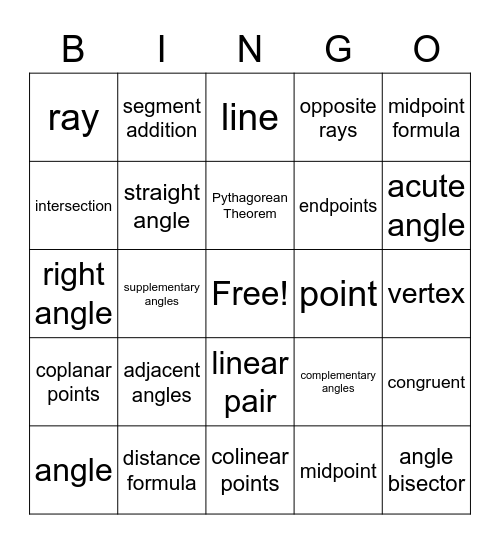Geometry Tools BingoThis bingo card has a free space and 29 words: angle, congruent, supplementary angles, plane, ray, line, straight angle, adjacent angles, point, distance formula, vertex, angle bisector, colinear points, midpoint, acute angle, segment bisector, linear pair, coplanar points, midpoint formula, right angle, complementary angles, vertical angles, endpoints, intersection, opposite rays, segment addition, congruent, Pythagorean Theorem and 90 degrees.

⚠ This card has duplicate items: congruent (2)

Play Online